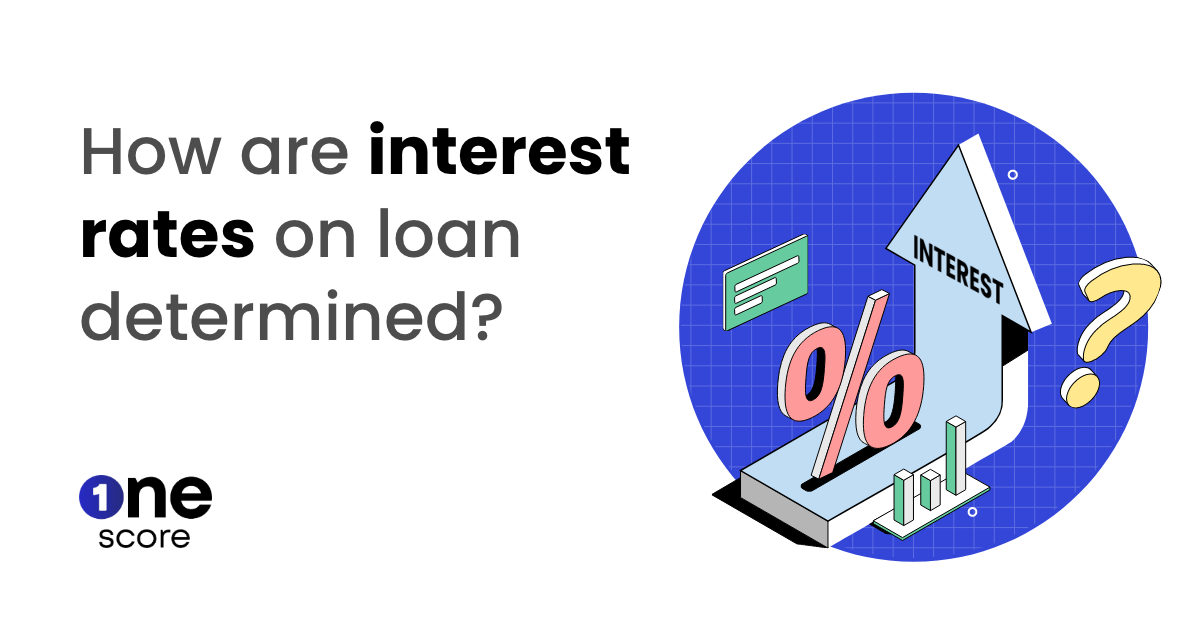# How to use an EMI Calculator?

Loans for all

An EMI calculator is a tool that helps borrowers determine their EMI amount for a loan. It takes into account the loan amount, interest rate, and loan tenure.Listen to the article here:

It's really important to handle your money well when you have to pay back a loan. One useful thing to help you with that is an EMI calculator. This tool is helpful when you want to buy something big like a house or a car and need a loan. The EMI calculator makes things easier by telling you how much you need to pay each month (your EMI). In this blog, we'll talk about EMI calculators, how to use them, why they're important, and what things can affect your loan EMI.

## What is an EMI?

EMI stands for Equated Monthly Installment. EMI is the monthly installment you pay towards your loan at a specified interest rate over the loan tenure. An EMI has two components – principal repayment and interest. During the initial years, a significant portion of the EMI consists of the interest amount. However, towards the end of loan tenure, the principal amount makes up for a major part of the EMI payment.

## What is an EMI Calculator?

An EMI calculator is a handy financial tool that helps individuals calculate their monthly loan installment with precision. It eliminates the need for complex manual calculations. By entering loan details, such as the loan amount, interest rate, and tenure, the calculator provides the borrower with the exact EMI amount they need to pay.

## How Does the EMI Calculator Work?

The EMI calculator uses a formula to compute the EMI based on the loan amount, interest rate, and tenure provided. It takes into account the reducing balance method, where the principal amount decreases with each payment made. This results in a lower interest payment over time. The formula used in the calculation is:

EMI = [P x R x (1+R)^N]/[(1+R)^N-1]

Where: P = Loan amount R = Monthly interest rate (annual interest rate divided by 12) N = Loan tenure in months

The EMI calculator utilizes this formula to provide an accurate EMI amount in a matter of seconds. For a quicker and simpler way to calculate EMIs, you can just keep the OneScore EMI Calculator handy.

## How to Use OneScore EMI Calculator:

Using an EMI calculator is a straightforward process. Here's a step-by-step guide:

Step 2: Click on “Check My Score” to move ahead.

Step 3: Enter your mobile number.

Step 4: Enter the OTP for a secure app experience.

Step 6: On the homepage, click on “Calculate EMI”.

Step 7: Select the loan type you want to calculate EMI, from Personal Loan/Home Loan/Auto Loan/Others.

Step 8: Input loan details: Enter the loan amount, interest rate, and loan tenure into the calculator. Ensure you have accurate information to obtain precise results.## Types of EMI Calculators:

• ### Home Loan EMI Calculator:

Specifically designed for home loans, this calculator helps determine the EMI for purchasing or constructing a house.

• ### Car Loan EMI Calculator:

Tailored for car loans, this calculator assists in estimating the monthly installment for buying a car.

• ### Personal Loan EMI Calculator:

This calculator aids in calculating the EMI for personal loans, allowing borrowers to plan their finances accordingly.

• ## Factors Affecting Loan EMI:

• ### Loan Amount:

The principal amount borrowed plays a significant role in determining the EMI. Higher loan amounts result in higher EMIs, assuming all other factors remain constant.

• ### Interest Rate:

The interest rate charged by the lender affects the EMI. Higher interest rates lead to larger EMIs, while lower rates result in smaller EMIs>

• ### Loan Tenure:

The tenure or duration of the loan also impacts the EMI. Longer loan tenures result in smaller EMIs, while shorter tenures lead to larger EMIs.

• ### Prepayments:

Making prepayments or paying off the loan before the due date can impact the EMI. Prepayments lower the principal amount, which reduces the interest charged, resulting in a lower EMI.

1. ## EMI Calculator FAQs:

2. Is an EMI calculator accurate?
3. Yes, EMI calculators are highly accurate, provided that the inputs entered are precise.

4. Can I use an EMI calculator for any type of loan?
5. Yes, EMI calculators are versatile and can be used for home loans, car loans, personal loans, and other types of loans.

6. Is the EMI fixed throughout the loan tenure?
7. Yes, the EMI is fixed throughout the loan tenure, and the borrower is required to pay the same amount each month until the loan is fully repaid.

8. Can I change my EMI amount during the loan tenure?
9. No, the EMI amount is fixed, and you cannot change it during the loan tenure. However, you can opt for loan prepayments, which can help you reduce the principal amount and lower the EMI.

## Conclusion:

In summary, an EMI calculator is an essential financial tool that can help individuals plan and manage their loans effectively. By providing accurate EMI calculations, borrowers can make informed decisions and ensure timely loan repayments. When using an EMI calculator, it is essential to input accurate information to obtain precise results. By understanding the factors that affect the EMI and the different types of EMI calculators available, borrowers can make informed financial decisions and manage their loans effectively.

More articlesLoans for all   |   May 11, 2023Loans for all   |   May 04, 2023Loans for all   |   Apr 21, 2023Loans for all   |   Apr 19, 2023Loans for all   |   Apr 11, 2023Loans for all   |   Apr 06, 2023

-   OneScore , May 16, 2023

Sharing is caring 😉